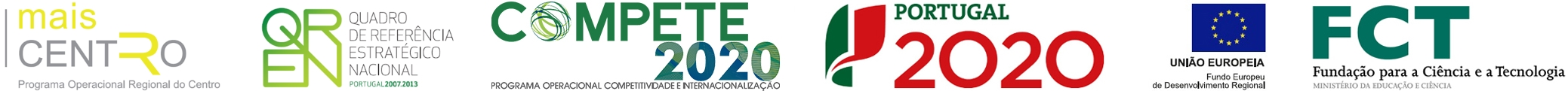Nonlinear dc electric-field dependence of the dielectric permittivity and cluster polarization of Sr1-xMnxTiO3 ceramics
 authors Tkach, A; Vilarinho, PM; Kholkin, AL nationality International journal JOURNAL OF APPLIED PHYSICS keywords STRONTIUM-TITANATE CERAMICS; SOFT PHONON MODES; SRTIO3; FERROELECTRICITY; SR1-XCAXTIO3; RANDOMNESS; TRANSITION; DEVICES abstract The dc electric-field dependence of the dielectric constant epsilon(')(E) in Sr1-xMnxTiO3 (x=0.005-0.02) ceramics is studied in the temperature range from 10 to 125 K, i.e., around the epsilon(')(T) peak temperature T-max. Results are analyzed using equations derived analytically from the implicit equations of the Landau-Ginzburg-Devonshire (LGD) theory. Corrections due to cluster contribution attributed to the reorientation of random-field-induced polar nanometer-scale regions turn out to be important, if not decisive, for a reliable description of epsilon(')(E). So, it is found that a combined equation including the Langevin-type cluster term, i.e., epsilon(')(L)(E)=(P0L3)-L-2/(2k(B)T epsilon(0)[cosh((P0LE)-E-3/2k(B)T)](2)) is the most suited to describe the dc electric-field dependence of the dielectric constant around T-max. Based on the fitting of the epsilon(')(E) data, the cluster polarization P-0 reaches values as high as similar to 0.4 mu C/cm(2) with the cluster size L of 11 +/- 4.5 nm. Thus, the existence in the Sr1-xMnxTiO3 system of polar clusters with nanometer-scale size is consistent with epsilon(')(E) data and their contribution to the permittivity at E=0 and temperatures close to T-m is estimated to be up to 30%. In addition, besides inducing the peak in epsilon(T), the formation of polar nanoregions is beneficial to the high relative electric-field tunability of the dielectric constant n(r)=[epsilon(')(0)-epsilon(')(E)]/epsilon(')(0)similar to 70% as well as high communication quality factor K=[epsilon(')(0)/epsilon(')(E)-1](2)/[epsilon(')(0)/epsilon(')(E)tan delta(0)tan delta(E)]similar to 10 000 under 20 kV/cm at 10 kHz. publisher AMER INST PHYSICS issn 0021-8979 year published 2007 volume 101 issue 8 digital object identifier (doi) 10.1063/1.2717874 web of science category Physics, Applied subject category Physics unique article identifier WOS:000246072200115
ciceco authors
impact metrics
 journal analysis (jcr 2019): journal impact factor 2.286 5 year journal impact factor 2.138 category normalized journal impact factor percentile 54.87
 dimensions (citation analysis): altmetrics (social interaction):

Apoio